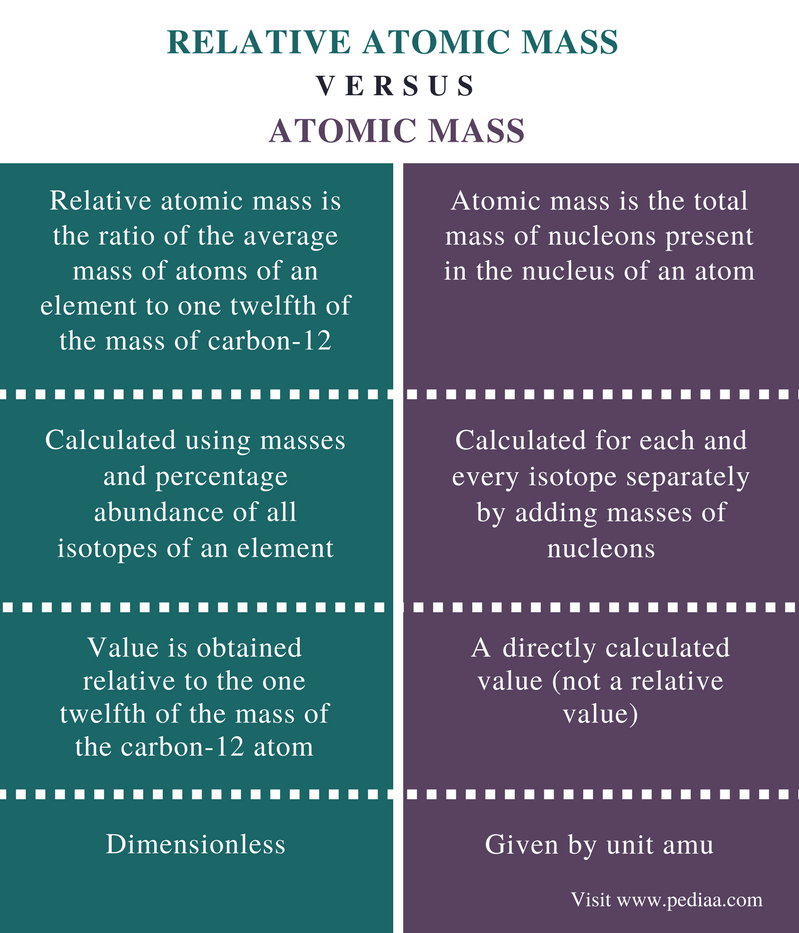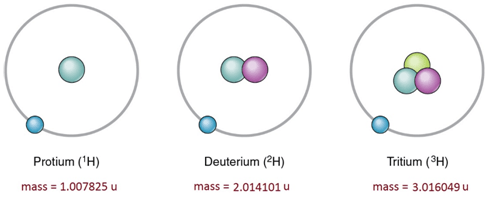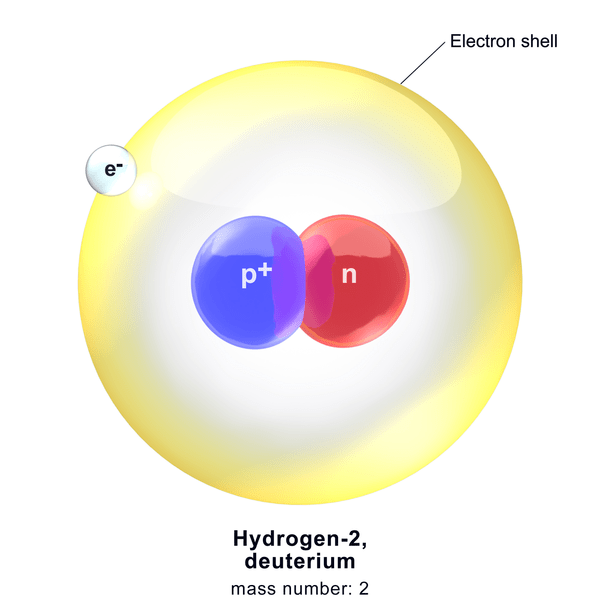# Difference Between Relative Atomic Mass and Atomic Mass

## Main Difference – Relative Atomic Mass vs Atomic Mass

Atoms are the basic units of matter. Scientific discoveries have revealed that an atom can be further divided into subatomic particles: electrons, protons, and neutrons. It was also discovered that an atom has a complex structure with a central core, named nucleus and electrons moving around this nucleus. The nucleus contains protons and neutrons. Relative atomic mass and atomic mass are two chemical terms that are used to express the mass of an atom. The main difference between relative atomic mass and atomic mass is that relative atomic mass is the ratio of the average mass of atoms of an element to one twelfth of the mass of carbon-12 whereas atomic mass is the total mass of nucleons present in the nucleus of an atom.

### Key Areas Covered

1. What is Relative Atomic Mass
– Definition, Calculation, Example
2. What is Atomic Mass
– Definition, Calculation, Example
3. What is the Difference Between Relative Atomic Mass and Atomic Mass
– Comparison of Key Differences

Key Terms: Atom, Atomic Mass, Electrons, Neutrons, Nucleus, Protons, Relative Atomic Mass## What is Relative Atomic Mass

Relative atomic mass is the ratio of the average mass of atoms of an element to one twelfth of the mass of carbon-12. One twelfth of the mass of an atom of carbon-12 is named as atomic mass unit (1 amu or 1 u). Hence, the relative atomic mass of carbon-12 is 12 amu.

Relative atomic mass = average mass of an atom/mass of carbon-12 x (1/12)

The average mass of an atom is calculated using the masses of different isotopes of an element and their abundance. The value of one twelfth of mass of carbon-12 isotope is 1.66054 x 10-18g. It is equal to 1 u or one unified atomic mass unit. Let’s consider a hydrogen atom and calculate the relative atomic mass.

### Relative Atomic Mass of Hydrogen

First, we need to find the average mass of a hydrogen atom.

 Isotope Abundance (%) Mass (u) Hydrogen-1 99.98 1.007825 Hydrogen-2 0.02 2.014101 Hydrogen-3 Trace ‎3.016049Figure 1: Isotopes of Hydrogen

Average mass of hydrogen = (1.007825 u x 99.98%) + (2.014101 u x0.02 %)
= (1.007623 + 0.0000402) u
= 1.0076632 u

The value of one twelfth of the mass of carbon-12 isotope is 1 u.

Therefore,

Relative atomic mass  = average mass of an atom/mass of carbon-12 x (1/12)
= 1.0076632 u / 1 u
= 1.0076632

Here, the mass of tritium was not included in the calculation because its abundance is trace in the environment and is negligible. The final value is dimensionless because it is a relative value.

## What is Atomic Mass

Atomic mass is the total mass of nucleons present in the nucleus of an atom. A nucleon is either a proton or a neutron. Hence, atomic mass is the total mass of the proton and neutrons present in the nucleus. Although electrons are also present in atoms, the mass of electrons is not used in the calculations because electrons are so small and have a negligible mass when compared to protons and neutrons.

Unlike in relative atomic mass, here we calculate the mass of each and every atom without calculating any average value. Therefore, we get different values for atomic masses of different isotopes. That is because the number of nucleons present in isotopes of the same element is different from each other.

Let us consider the same example as for relative atomic mass; hydrogen.

Atomic Mass of Hydrogen-2

The atomic mass of hydrogen-2 (Deuterium) isotope is calculated as follows.

The number of protons in the nucleus     = 1
The number of neutrons in the nucleus   = 1
Atomic mass of hydrogen                            = (1 amu +1 amu)
= 2 amuFigure 2: Structure of Deuterium

The atomic mass is given by the unit amu (atomic mass units). One protons or a neutron has a mass of 1 amu.

## Difference Between Relative Atomic Mass and Atomic Mass

### Definition

Relative Atomic Mass: Relative atomic mass is the ratio between the average mass of atoms of an element to one twelfth of the mass of carbon-12.

Atomic Mass: Atomic mass is the total mass of nucleons present in the nucleus of an atom.

### Isotopes

Relative Atomic Mass: Relative atomic mass is calculated using the masses and the percentage abundance of all the isotopes of an element.

Atomic Mass: Atomic mass is calculated for each and every isotope separately by adding masses of nucleons.

### Value

Relative Atomic Mass: The value of Relative atomic mass is obtained relative to the one twelfth of the mass of the carbon-12 atom.

Atomic Mass: The value of atomic mass is a directly calculated value (not a relative value).

### Unit

Relative Atomic Mass: Relative atomic mass is dimensionless because it is a relative value.

Atomic Mass: Atomic mass is given by unit amu.

### Conclusion

Atomic mass and relative atomic mass are two important chemical terms. Although they sound similar, the concepts are different. The main difference between relative atomic mass and atomic mass is that relative atomic mass is the ratio of the average mass of atoms of an element to one twelfth of the mass of carbon-12 whereas atomic mass is the total mass of nucleons present in the nucleus of an atom.

##### References:

1. “Relative atomic mass.” Wikipedia, Wikimedia Foundation, 26 Oct. 2017, Available here.
2. “Atomic Mass.” Merriam-Webster, Merriam-Webster, Available here.
3. wikiHow. “How to Calculate Atomic Mass.” WikiHow, WikiHow, 5 Oct. 2017, Available here.

##### Image Courtesy:

1. “204 Isotopes of Hydrogen-01” By OpenStax College – Anatomy & Physiology, Connexions Web site, Jun 19, 2013. (CC BY 3.0) via Commons Wikimedia
2. “Blausen 0527 Hydrogen-2 Deuterium” By BruceBlaus – Own work (CC BY 3.0) via Commons Wikimedia